The Queer BookshelfThe Queer Bookshelf::Under Construction::Secret Passages Lead to a Shack Where Queeks Learn to Their Hearts' Content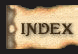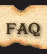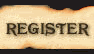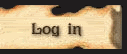Share

# Zorita's Remedial MathAuthorMessage
Zorita
FounderSubject: Zorita's Remedial MathThu 11 Oct 2018, 14:56 Covering all dese topics:Mini-lessons Being Worked On (WO)Mini-lessons CompletedSort by: Difficulty levelMost recent date: 10.8.2018Titles:Complementary vs Supplementary Angles Composite Numbers vs Prime Numbers Decimal Numbers: The Basics Fractions: The Basics Using Division Tables to Solve Division Problems Using Rounding as an Estimating Technique Algebra Terms: The Basics Calculating the Percentage of a Total When the Portion and the Total Are Known Values Coefficients: The Basics Congruent Angles Creating Equations that Solve Word Problems Dependent vs Independent Variables Determining the Mean of a Data Set Determining the Sign of a Product with One or More Negative Factors Equations: The Basics Exponential Expressions: The Basics Factoring Polynomials: The Basics Factoring Quadratics Finding the Reciprocal of a Fraction Finding the Value of Numbers with Negative Exponents Function Tables: The Basics Like Terms vs Unlike TermsLines vs Line Segments vs Rays Multiplicity in Prime Factorization Numeric Patterns Placing Rational Numbers on a Number Line Raising Fractions to Exponential Powers Range of a Data Set Rational Numbers vs Irrational Numbers Ratios: The Basics Remainders: The Basics Setting up the Equation in a Word Problem Shortcuts for Factoring Quadratics Simplifying Equations by Clearing the Fractions Simplifying Expressions by Combining Like Terms The Angles Formed by Transversals The Degree of a Term or Polynomial The Pythagorean Theorem Translating Word Problem Sentences into Algebraic Expressions Using Division to Isolate the Variable in a Multiplication Equation Vertical Angles Using the Substitution Method to Solve Systems of Linear Equations Calculating the Value of the Number Represented by a Percentage of Any Total Computing Compound Interest Determining the Number of Permutations for All the Items in a Data Set Dividing Polynomials by Monomials Factorials Finding the Area of a Rhombus Finding the Difference between Two Perfect Squares Finding the Slope of a Line Finding the Slope of a Perpendicular Line Finding the Surface Area of a Sphere Finding the Variable in Equations Made Up of Proportional Fractions Function Tables: Advanced Graphing a Linear Function Isolating the Variable in an Equation When the Coefficient is a Fraction Placing Irrational Numbers on a Number Line Prime Factorization Quartiles: The Basics Solving Inequalities Sorting Data with Venn Diagrams The Differences Between Sequences and Series Venn Diagrams: The Basics Verifying the Proportionality of Fractions through Cross Multiplication Writing Numbers in Scientific Notation Evaluating Composition Functions When the Value of the Variable is Given Zorita provide proof did da work... Zorita overwhelmed!Last edited by Zorita on Thu 11 Oct 2018, 15:46; edited 1 time in total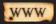# Zorita's Remedial MathPage 1 of 1

# Similar topics» Math Problems and Giving Up

Permissions in this forum:You cannot reply to topics in this forum